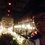# Perimeter-like Grids

Page 9

## Perimeter and Semiperimeter

In general, the perimeter of a $a\times b$ grid is $2a+2b$, the semiperimeter of a $a\times b$ grid is $a+b$.

For example, the perimeter of a $3\times 5$ grid is 16, its semiperimeter is 8.

## Perimeter-like Grids

If two grids have the same perimeter, then these 2 grids are "perimeter-like grids".For example, $5\times 2$ grid and $3\times 4$ grid are perimeter-like grids.

## Maximum number of unit squares

Suppose there's a grid, $a+b=10$, if the number of unit squares in the grid reaches its maximum, then what is the value of $a$ and $b$?

Let's write out the possibilities: $1\times9,~~~~2\times8,~~~~3\times7,~~~~4\times6,~~~~5\times5,~~~~6\times4,~~~~7\times3,~~~~8\times2,~~~~9\times1$ The corresponding numbers of unit squares are: $9,~~~~~~16,~~~~~~21,~~~~~~24,~~~~~~25,~~~~~~24,~~~~~~21,~~~~~~16,~~~~~~9$ We see that when $a=b=5$, then the number of unit squares reaches its maximum.

We say that the maximum number of unit squares it can form when $a+b=10$ is 25.

What if $a+b$ is an odd number? Let's try this out...

Suppose $a+b=9$, the possibilities are: $1\times8,~~~~2\times7,~~~~3\times6,~~~~4\times5,~~~~5\times4,~~~~6\times3,~~~~7\times2,~~~~8\times1$ The corresponding numbers of unit squares are: $8,~~~~~~14,~~~~~~18,~~~~~~20,~~~~~~20,~~~~~~18,~~~~~~14,~~~~~~8$ We see that when $a=b\pm1$, then the number of unit squares reaches its maximum.

We say that the maximum number of unit squares it can form when $a+b=9$ is 20.

## The Greatest Rule

If $a+b$ is a fixed even number, then when $a=b$, the number of unit squares it can form in the grid will reach its maximum.

Another way of thinking about this is among all the perimeter-like grids which $a+b$ is even, then the grids which has the property of $a=b$ will have the most number of unit squares.

If $a+b$ is a fixed odd number, then when $a=b\pm1$, the number of unit squares it can form in the grid will reach its maximum.

Another way of thinking about this is among all the perimeter-like grids which $a+b$ is odd, then the grids which has the property of $a=b\pm1$ will have the most number of unit squares.

Proof:
Consider $a+b$ is even. Suppose $a+b=2k$, $a=k+x$, $b=k-x$ ($k$ is a positive integer while $x$ is an integer). The number of unit squares in the grid is \begin{aligned} a\times b&=(k+x)(k-x) \\&=k^2-x^2 \end{aligned} Since $x^2\geqslant 0$, to maximize the value of $k^2-x^2$, $x$ must be equal to 0, thus $a=b=k$.
Consider $a+b$ is odd. Suppose $a+b=2k+1$, $a=k+x$, $b=k+1-x$. The number of unit squares in the grid is \begin{aligned} a\times b&=(k+x+1)(k-x) \\&=k^2+k-x^2-x \\&=k^2+k-x(x-1) \end{aligned} Since $x(x-1)\geqslant 0$, to maximize the value of $k^2+k-x(x-1)$, $x$ must be equal to 0 or 1, thus $a=k$, $b=k+1$ or $a=k+1$, $b=k$, $a=b\pm1$.

Generalization:

If $a+b$ is a fixed even number, then when $a=b=k$, the number of unit squares in the grid will reach its maximum, which is $ab=k^2=\left (\frac{a+b}{2} \right )^2$.

$\bullet$ Hence if $a+b$ is even, the maximum number of unit squares it can form is $\left (\frac{a+b}{2} \right )^2$.

If $a+b$ is a fixed odd number, then when $a=k+1$, $b=k$ or when $a=k$, $b=k+1$, the number of unit squares in the grid will reach its maximum, which is $ab=k(k+1)=\left (\frac{2k}{2} \right )\left (\frac{2k+2}{2} \right )=\frac{(a+b-1)(a+b+1)}{4}$.

$\bullet$ Hence if $a+b$ is odd, the maximum number of unit squares it can form is $\frac{(a+b-1)(a+b+1)}{4}$.

## Worked examples

If $a+b=14$, what is the maximum number of unit squares it can form?

Solution:

Since 14 is even, according to the greatest rule, the maximum number of unit squares it can form is $\left( \frac{a+b}{2} \right)^2=7^2=49$.

If $a+b=19$, what is the maximum number of unit squares it can form?

Solution:

Since 19 is odd, according to the greatest rule, the maximum number of unit squares it can form is $\frac{(a+b-1)(a+b+1)}{4}=\frac{18\times 20}{4}=90$.

This is one part of Grids and Quadrilaterals.Note by Kenneth Tan
5 years, 6 months ago

This discussion board is a place to discuss our Daily Challenges and the math and science related to those challenges. Explanations are more than just a solution — they should explain the steps and thinking strategies that you used to obtain the solution. Comments should further the discussion of math and science.

When posting on Brilliant:

• Use the emojis to react to an explanation, whether you're congratulating a job well done , or just really confused .
• Ask specific questions about the challenge or the steps in somebody's explanation. Well-posed questions can add a lot to the discussion, but posting "I don't understand!" doesn't help anyone.
• Try to contribute something new to the discussion, whether it is an extension, generalization or other idea related to the challenge.

MarkdownAppears as
*italics* or _italics_ italics
**bold** or __bold__ bold
- bulleted- list
• bulleted
• list
1. numbered2. list
1. numbered
2. list
Note: you must add a full line of space before and after lists for them to show up correctly
paragraph 1paragraph 2

paragraph 1

paragraph 2

[example link](https://brilliant.org)example link
> This is a quote
This is a quote
    # I indented these lines
# 4 spaces, and now they show
# up as a code block.

print "hello world"
# I indented these lines
# 4 spaces, and now they show
# up as a code block.

print "hello world"
MathAppears as
Remember to wrap math in $$ ... $$ or $ ... $ to ensure proper formatting.
2 \times 3 $2 \times 3$
2^{34} $2^{34}$
a_{i-1} $a_{i-1}$
\frac{2}{3} $\frac{2}{3}$
\sqrt{2} $\sqrt{2}$
\sum_{i=1}^3 $\sum_{i=1}^3$
\sin \theta $\sin \theta$
\boxed{123} $\boxed{123}$